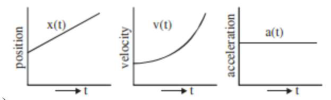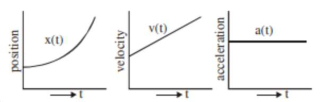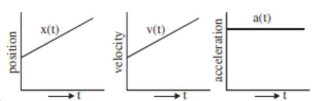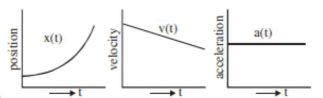# The position, velocity and acceleration of a particle moving with a constant

Question:

The position, velocity and acceleration of a particle moving with a constant

acceleration can be represented by:

1. (1)2. (2)3. (3)4.Correct Option: 2,

Solution:

Option (2) represent correct graph for particle moving with constant acceleration, as for constant acceleration velocity time graph is straight line with positive slope and $x-\operatorname{tg}$ graph should be an opening upward parabola.